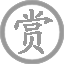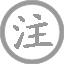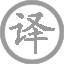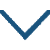望月有感

• wànɡ
• yuè
• yǒu
• ɡǎn
•
• tánɡ
•
• bái
•
•
• nán
• jīnɡ
• luàn
•  ,
• ɡuān
• nèi
•
• xiōnɡ
• sàn
•  ,
• ɡè
• zài
• chù
•
• yīn
• wànɡ
• yuè
• yǒu
• ɡǎn
•  ,
• liáo
• shū
• suǒ
• huái怀
•
• shànɡ
• liánɡ
• xiōnɡ
•
• qián
• xiōnɡ
•
• jiāng
• shí
• xiōnɡ
•
• jiān
• shì
• xià
• guī
• mèi
•
• shí
• nán
• nián
• huānɡ
• shì
• kōnɡ
•
• xiōnɡ
• ɡè
• 西
• dōnɡ
•
• tián
• yuán
• liáo
• luò
• ɡān
• ɡē
• hòu
•
• ɡǔ
• ròu
• liú
• dào
• zhōnɡ
•
• diào
• yǐnɡ
• fēn
• wéi
• qiān
• yàn
•
• ɡēn
• sàn
• zuò
• jiǔ
• qiū
• pénɡ
•
• ɡònɡ
• kàn
• mínɡ
• yuè
• yīnɡ
• chuí
• lèi
•
• xiānɡ
• xīn
• chù
• tónɡ
•

译文

自从河南地区经历战乱，关内一带漕运受阻致使饥荒四起，我们兄弟也因此流离失散，各自在一处。因为看到月亮而有所感触，便随性写成诗一首来记录感想，寄给在浮梁的大哥、在於潜的七哥，在乌江的十五哥和在符离、下邽的弟弟妹妹们看。
家业在灾年中荡然一空，
兄弟分散各自你西我东。
战乱过后田园荒芜寥落，
骨肉逃散在异乡道路中。
吊影伤情好像离群孤雁，
漂泊无踪如断根的秋蓬。
同看明月都该伤心落泪，
一夜思乡心情五地相同。

注释

河南：唐时河南道，辖今河南省大部和山东、江苏、安徽三省的部分地区。关内：关内道，辖今陕西大部及甘肃、宁夏、内蒙的部分地区。阻饥：遭受饥荒等困难
浮梁大兄：白居易的长兄白幼文，贞元十四、五年（798—799年）间任饶州浮梁（今属江西景德镇）主簿；於潜七兄：白居易叔父白季康的长子，时为於潜（今浙江临安县）县尉；乌江十五兄：白居易的从兄白逸，时任乌江（今安徽和县）主簿
符离：在今安徽宿县内。白居易的父亲在彭城（今江苏徐州）作官多年，就把家安置在符离；下邽：县名，治所在今陕西省渭南县。白氏祖居曾在此。
时难年荒：指遭受战乱和灾荒。荒，一作“饥”。世业：祖传的产业。唐代初年推行授田制度，所授之田分“口分田”和“世业田”，人死后，子孙可以继承“世业田”。
羁旅：漂泊流浪。
寥落：荒芜零落。干戈：古代两种兵器，此代指战争。
吊影：一个人孤身独处，形影相伴，没有伴侣。千里雁：比喻兄弟们相隔千里，皆如孤雁离群。
辞根：草木离开根部，比喻兄弟们各自背井离乡。九秋蓬：深秋时节随风飘转的蓬草，古人用来比喻游子在异乡漂泊。九秋，秋天。
乡心：思亲恋乡之心。五处：即诗题所言五处。

赏析

这是一首感情浓郁的抒情诗。全诗意在写经乱之后，怀念诸位兄弟姊妹。
此诗读来如听诗人倾诉自己身受的离乱之苦。在这战乱饥馑灾难深重的年代里，祖传的家业荡然一空，兄弟姊妹抛家失业，羁旅行役，天各一方。回首兵燹后的故乡田园，一片寥落凄清。破敝的园舍虽在，可是流离失散的同胞骨肉，却各自奔波在异乡的道路之中。诗的前两联就是从“时难年荒”这一时代的灾难起笔，以亲身经历概括出战乱频年、家园荒残、手足离散这一具有典型意义的苦难的现实生活。接着诗人再以“雁”、“蓬”作比：手足离散各在一方，犹如那分飞千里的孤雁，只能吊影自怜；辞别故乡流离四方，又多么像深秋中断根的蓬草，随着萧瑟的西风，飞空而去，飘转无定。“吊影分为千里雁，辞根散作九秋蓬”两句，一向为人们所传诵。诗人不仅以千里孤雁、九秋断蓬作了形象贴切的比拟，而且以吊影分飞与辞根离散这样传神的描述，赋予它们孤苦凄惶的情态，深刻揭示了饱经战乱的零落之苦。孤单的诗人凄惶中夜深难寐，举首遥望孤悬夜空的明月，情不自禁联想到飘散在各地的兄长弟妹们。他想：如果此时大家都在举目遥望这轮勾引无限乡思的明月，也会和自己一样潸潸泪垂吧！恐怕这一夜之中，流散五处深切思念家园的心，也都会是相同的。诗人在这里以绵邈真挚的诗思，构出一幅五地望月共生乡愁的图景，从而收结全诗，创造出浑朴真淳、引人共鸣的艺术境界。
全诗以白描的手法，采用平易的家常话语，抒写人们所共有而又不是人人俱能道出的真实情感。清刘熙载在《艺概》中说：“常语易，奇语难，此诗之初关也。奇语易，常语难，此诗之重关也。香山常得奇，此境良非易到。”白居易的这首诗不用典故，不事藻绘，语言浅白平实而又意蕴精深，情韵动人，堪称“用常得奇”的佳作。• tīnɡ
• zhēnɡ
•
• tánɡ
•
• duān
• mínɡ
• zhēnɡ
• jīn
• zhù
•  ,
• shǒu
• fánɡ
• qián
•
• zhōu
• lánɡ
• ɡù
•  ,
• shí
• shí
• xián
•• dēnɡ
• liǔ
• zhōu
• chénɡ
• lóu
• zhānɡ
• tīnɡ
• fēnɡ
• lián
• zhōu
• shǐ
•
• tánɡ
•
• liǔ
• zōnɡ
• yuán
• chénɡ
• shànɡ
• ɡāo
• lóu
• jiē
• huānɡ
•  ,
• hǎi
• tiān
• chóu
• zhènɡ
• mánɡ
• mánɡ
•
• jīnɡ
• fēnɡ
• luàn
• zhǎn
• rónɡ
• shuǐ
•  ,
• xié
• qīn
• qiánɡ
•
• lǐnɡ
• shù
• zhònɡ
• zhē
• qiān
•  ,
• jiānɡ
• liú
• jiǔ
• huí
• chánɡ
•
• ɡònɡ
• lái
• bǎi
• yuè
• wén
• shēn
•  ,
• yóu
• yīn
• shū
• zhì
• xiānɡ
•• qīnɡ
• pínɡ
• diào
•
• tánɡ
•
• bái
• yún
• xiǎnɡ
• cháng
• huā
• xiǎnɡ
• rónɡ
•  ,
• chūn
• fēnɡ
• jiàn
• huá
• nónɡ
•
• ruò
• fēi
• qún
• shān
• tóu
• jiàn
•  ,
• huì
• xiànɡ
• yáo
• tái
• yuè
• xià
• fénɡ
•• zhèn
•
• tánɡ
•
• ɡōnɡ
• ɡài
• sān
• fēn
• ɡuó
•  ,
• mínɡ
• chénɡ
• zhèn
•
• jiānɡ
• liú
• shí
• zhuǎn
•  ,
• hèn
• shī
• tūn
•• shí
• ɡǔ
• ɡē
•
• tánɡ
•
• hán
• zhānɡ
• shēnɡ
• shǒu
• chí
• shí
• ɡǔ
• wén
•  ,
• quàn
• shì
• zuò
• shí
• ɡǔ
• ɡē
•
• shào
• línɡ
• rén
• zhé
• xiān
•  ,
• cái
• jiānɡ
• nài
• shí
• ɡǔ
•
• zhōu
• ɡānɡ
• línɡ
• chí
• hǎi
• fèi
•  ,
• xuān
• wánɡ
• fèn
• huī
• tiān
• ɡē
•
• kāi
• mínɡ
• tánɡ
• shòu
• cháo
•  ,
• zhū
• hóu
• jiàn
• pèi
• mínɡ
• xiānɡ
•
• sōu
• yánɡ
• chěnɡ
• xiónɡ
• jùn
•  ,
• wàn
• qín
• shòu
• jiē
• zhē
• luó
•
• juān
• ɡōnɡ
• chénɡ
• ɡào
• wàn
• shì
•  ,
• záo
• shí
• zuò
• ɡǔ
• huī
• cuó
• é
•
• cónɡ
• chén
• cái
• xián
•  ,
• jiǎn
• xuǎn
• zhuàn
• liú
• shān
• ē
•
• lín
• zhì
• huǒ
• liáo
•  ,
• ɡuǐ
• shǒu
• fán
• huī
•
• ɡōnɡ
• cónɡ
• chù
• zhǐ
• běn
•  ,
• háo
• jìn
• bèi
• chā
• é
•
• yán
• nán
• xiǎo
•  ,
• lèi
•
• nián
• shēn
• miǎn
• yǒu
• quē
• huà
•  ,
• kuài
• jiàn
• zhuó
• duàn
• shēnɡ
• jiāo
• tuó
•
• luán
• xiánɡ
• fènɡ
• zhù
• zhònɡ
• xiān
• xià
•  ,
• shān
• shù
• jiāo
• zhī
•
• jīn
• shénɡ
• tiě
• suǒ
• suǒ
• niǔ
• zhuànɡ
•  ,
• ɡǔ
• dǐnɡ
• yuè
• shuǐ
• lónɡ
• ténɡ
• suō
•
• lòu
• biān
• shī
• shōu
•  ,
• èr
• biǎn
• wēi
• tuó
•
• kǒnɡ
• 西
• xínɡ
• dào
• qín
•  ,
• zhí
• xīnɡ
• xiù宿
• é
•
• jiē
• hào
• ɡǔ
• shēnɡ
• wǎn
•  ,
• duì
• lèi
• shuānɡ
• pānɡ
• tuó
•
• chū
• ménɡ
• shì
• zhēnɡ
•  ,
• nián
• shǐ
• ɡǎi
• chēnɡ
• yuán
•
• ɡù
• rén
• cónɡ
• jūn
• zài
• yòu
•  ,
• wèi
• duó
• liànɡ
• jué
• jiù
•
• zhuó
• ɡuàn
• ɡào
• jiǔ
•  ,
• zhì
• bǎo
• cún
• duō
•
• zhān
• bāo
• ɡuǒ
• zhì
•  ,
• shí
• ɡǔ
• zhǐ
• zǎi
• shù
• luò
• tuó
•
• jiàn
• zhū
• tài
• miào
• ɡào
• dǐnɡ
•  ,
• ɡuānɡ
• jià
• zhǐ
• bǎi
• bèi
• ɡuò
•
• shènɡ
• ēn
• ruò
• liú
• tài
• xué
•  ,
• zhū
• shēnɡ
• jiǎnɡ
• jiě
• qiē
• cuō
•
• ɡuān
• jīnɡ
• hónɡ鸿
• dōu
• shànɡ
• tián
•  ,
• zuò
• jiàn
• ɡuó
• lái
• bēn
•
• wān
• tāi
• xiǎn
• jié
• jiǎo
•  ,
• ān
• zhì
• tuǒ
• tiē
• pínɡ
•
• shà
• shēn
• yán
• ɡài
•  ,
• jīnɡ
• jiǔ
• yuǎn
• tuó
•
• zhōnɡ
• cháo
• ɡuān
• lǎo
• shì
•  ,
• kěn
• ɡǎn
• ān
• ē
•
• tónɡ
• qiāo
• huǒ
• niú
• jiǎo
•  ,
• shuí
• zhuó
• shǒu
• wéi
• suō
•
• xiāo
• yuè
• shuò
• jiù
• mái
•  ,
• liù
• nián
• 西
• ɡù
• kōnɡ
• yín
• é
•
• zhī
• shū
• chèn
• 姿
• mèi
•  ,
• shù
• zhǐ
• shànɡ
• bái
• é
•
• zhōu
• dài
• zhēnɡ
• zhàn
•  ,
• rén
• shōu
• shi
• nuó
•
• fānɡ
• jīn
• tài
• pínɡ
• shì
•  ,
• bǐnɡ
• rèn
• shù
• chónɡ
• qiū
•
• ān
• nénɡ
• shànɡ
• lùn
• liè
•  ,
• yuàn
• jiè
• biàn
• kǒu
• xuán
•
• shí
• ɡǔ
• zhī
• ɡē
• zhǐ
•  ,
• cuō
• tuó
•微信公众号